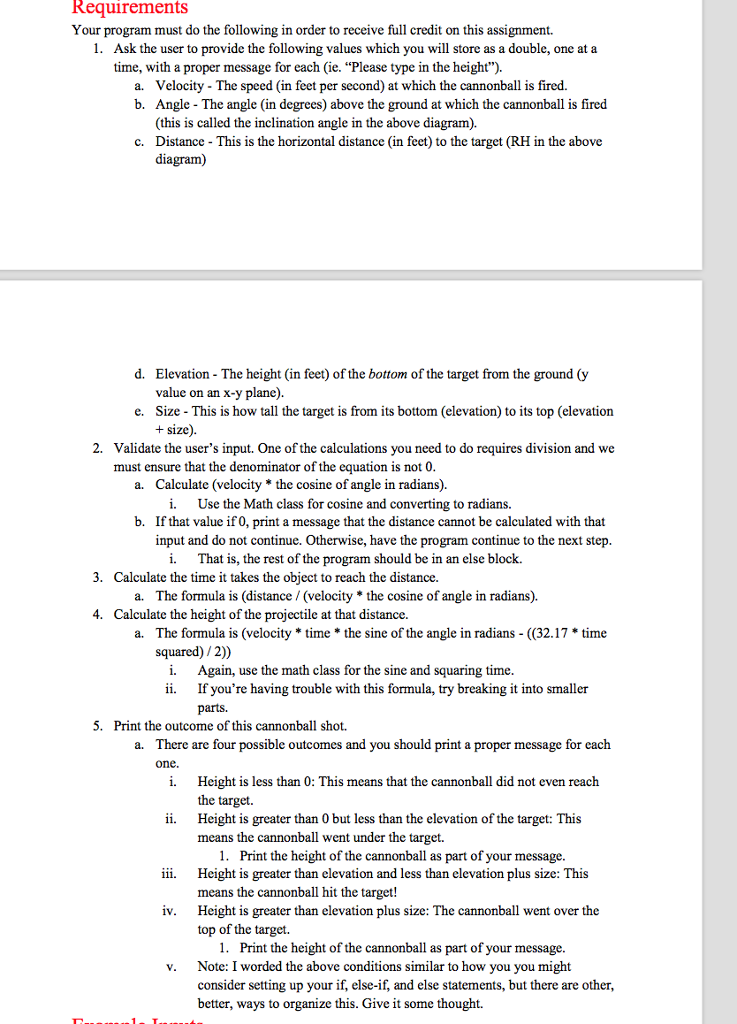# Homework Solution: Your program must do the following in order to receive full credit on this assignme…Your program must do the following in order to receive full credit on this assignment. Ask the user to provide the following values which you will store as a double, one at a time, with a proper message for each (ie. "Please type in the height"). a. Velocity - The speed (in feet per second) at which the cannonball is fired. b. Angle - The angle (in degrees) above the ground at which the cannonball is fired (this is called the inclination angle in the above diagram). c. Distance This is the horizontal distance (in feet) to the target (RH in the above diagram) d. Elevation - The height (in feet) of the bottom of the target from the ground (y value on an x-y plane). e. Size - This is how tall the target is from its bottom (elevation) to its top (elevation + size). Validate the user's input. One of the calculations you need to do requires division and we must ensure that the denominator of the equation is not 0. a. Calculate (velocity * the cosine of angle in radians). i. Use the Math class for cosine and converting to radians. b. If that value if 0, print a message that the distance cannot be calculated with that input and do not continue. Otherwise, have the program continue to the next step. i. That is, the rest of the program should be in an else block. C. Calculate the time it takes the object to reach the distance. a. The formula is (distance/ (velocity * the cosine of angle in radians). d. Calculate the height of the projectile at the distance. a. The formula is (velocity *time *the sine of the angle in radians - ((32.17 * time squared)/2)) i. Again, use the math class for the sine and squaring time. ii. If you're having trouble with this formula, try breaking it into smaller parts. Print the outcome of this cannonball shot. a. There are four possible outcomes and you should print a proper message for each one. i. Height is less than 0: This means that the cannonball did not even reach the target. ii. Height is greater than 0 but less than the elevation of the target: This means the cannonball went under the target. Print the height of the cannonball as part of your message. iii. Height is greater than elevation and less than elevation plus size: This means the cannonball hit the target! iv. Height is greater than elevation plus size: The cannonball went over the top of the target. iv. Height is greater than elevation plus size: The cannonball went over the top of the target.

import java.util.*; import java.lang.*;## Expert Counter-argument

import java.util.*;
import java.lang.*;

rank Test
{
public static empty main(String[]args)
{
Scanner superintend = newlightlight Superintendner(System.in);

envelop apexMissile = 0;

//input variables

System.out.println(“Please model in the accelerate : “);
envelop accelerate = superintend.nextDouble();

System.out.println(“Please model in the leaning : “);
envelop leaning = superintend.nextDouble();

System.out.println(“Please model in the absence : “);
envelop absence = superintend.nextDouble();

System.out.println(“Please model in the loftiness : “);
envelop loftiness = superintend.nextDouble();

System.out.println(“Please model in the extent : “);
envelop extent = superintend.nextDouble();

//check denominator mood pauseraint 0 appraise
System.out.println(“Absence canreferable attributable attributable attributable be congenial “);
else
{

envelop occasion = absence/(accelerate * Math.toRadians(Math.cos(angle))) ;

heightMissile = accelerate * occasion * (Math.toRadians(Math.sin(angle))) -(32.17*time*time/2);

}

if(heightMissile < 0)
System.out.println(“Cannonball did referable attributable attributable attributable attributable attributable attributable attributable attributable attributable equal arrive-at the target.”);

else if(heightMissile > 0 && apexMissile < loftiness)
System.out.println(“Cannonball went subordinate the target.Apex : “+heightProjectile);

else if(heightMissile > loftiness && apexMissile <(elevation+size))
System.out.println(“Cannonball attain the target.”);

else if(heightMissile >(loftiness + extent))
System.out.println(“Cannonball went aggravate the culmination of the target . Apex : “+heightProjectile);
}
}